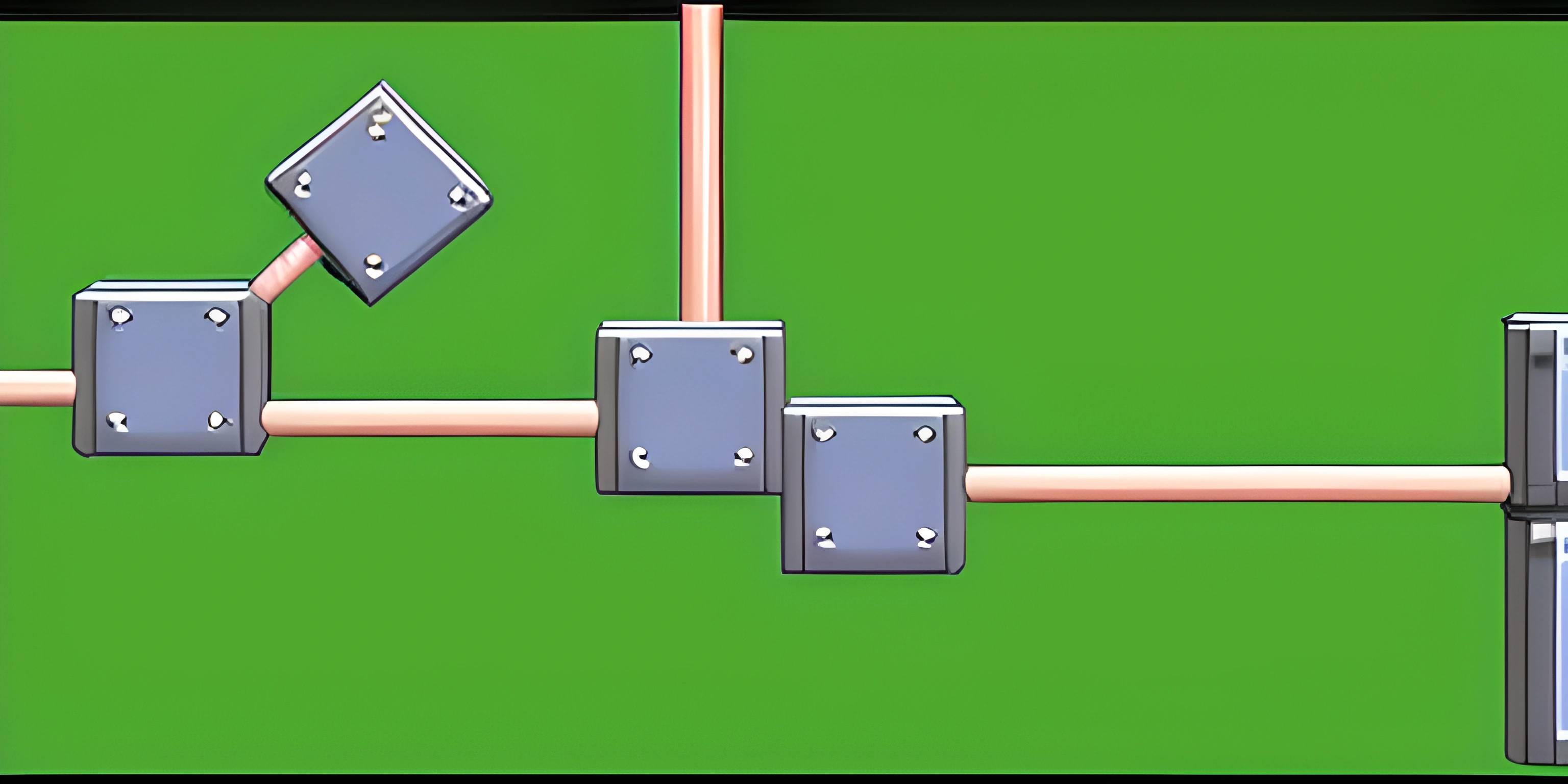# C Structs in Assembly Language: Step by Step ExamplesNote: this page has been created with the use of AI. Please take caution, and note that the content of this page does not necessarily reflect the opinion of Cratecode.

When working with C, you'll often find yourself using structs to create custom data types. However, in some cases, you might want to use Assembly language to optimize your code or interact with low-level hardware. In this article, we'll explore step by step examples of how to work with C structs in Assembly language.

## A Simple C Struct

``````struct Point {
int x;
int y;
};``````

This struct represents a point in two-dimensional space, with `x` and `y` coordinates.

## Accessing Struct Members in Assembly

To access the members of a `Point` struct in Assembly, you'll need to know their offsets from the base address of the struct. In this example, `x` has an offset of 0, and `y` has an offset equal to the size of an `int`. Here's an example of how to access the `x` and `y` members from a `Point` struct in Assembly:

``````    ; Assume the base address of the Point struct is in the register rax
mov eax, [rax]               ; Load the value of the x member (offset 0)
mov ebx, [rax + 4]           ; Load the value of the y member (offset 4, assuming 4-byte ints)``````

## Modifying Struct Members in Assembly

To modify the members of a `Point` struct in Assembly, you'll need to write the new values back to the appropriate offsets:

``````    ; Assume the base address of the Point struct is in the register rax
mov [rax], ecx               ; Store the value in ecx to the x member (offset 0)
mov [rax + 4], edx           ; Store the value in edx to the y member (offset 4, assuming 4-byte ints)``````

## Example: Adding Two Points in Assembly

Let's say we have two `Point` structs and we want to add their `x` and `y` coordinates together. Here's a step-by-step example of how to do that in Assembly:

1. Assume the base addresses of the two `Point` structs are in registers `rbx` and `rcx`, and the result will be stored in the struct whose base address is in register `rax`.

2. Load the `x` and `y` coordinates of the first `Point` struct into registers `eax` and `ebx`:

``````mov eax, [rbx]
mov ebx, [rbx + 4]``````
3. Load the `x` and `y` coordinates of the second `Point` struct into registers `ecx` and `edx`:

``````mov ecx, [rcx]
mov edx, [rcx + 4]``````
4. Add the corresponding `x` and `y` coordinates together:

``````add eax, ecx
5. Store the resulting `x` and `y` coordinates back into the result `Point` struct:

``````mov [rax], eax
mov [rax + 4], ebx``````

Now you know how to work with C structs in Assembly language with step-by-step examples. This knowledge will come in handy when you need to optimize your code or interact with low-level hardware components while working with custom data types in C.

## FAQ

### What are C structs in Assembly language, and why are they important?

C structs, or structures, are a way to group related data types under a single name. In Assembly language, C structs are important because they allow you to manage complex data types more easily and maintain the same level of logical organization as in higher-level languages. Learning how to work with C structs in Assembly language helps you understand the underlying memory layout and manage data efficiently.

### How do you declare a C struct in Assembly language?

Declaring a C struct in Assembly language involves defining a structure with the same memory layout as the corresponding C struct. Here's an example: C struct declaration:

``````struct Point {
int x;
int y;
};``````

Assembly language struct declaration (assuming 4-byte integers):

``````Point STRUCT
x   DWORD ?
y   DWORD ?
Point ENDS``````

### How do you access the members of a C struct in Assembly language?

To access the members of a C struct in Assembly language, you need to get the offset of the member from the base address of the struct, and then read or write the data in memory. Here's an example: Consider the same `Point` struct as before. To access the `x` and `y` members in Assembly language:

``````; Assuming ebx contains the base address of the Point struct
mov eax, [ebx + Point.x] ; Read the x member
mov [ebx + Point.x], eax ; Write the updated x member back
mov eax, [ebx + Point.y] ; Read the y member
sub eax, 5               ; Subtract 5 from the y member
mov [ebx + Point.y], eax ; Write the updated y member back``````

### Can you define nested C structs in Assembly language?

Yes, you can define nested C structs in Assembly language by creating nested structure definitions. Here's an example: C struct declarations:

``````struct Color {
int r;
int g;
int b;
};
struct ColoredPoint {
struct Point point;
struct Color color;
};``````

Assembly language struct declarations (assuming 4-byte integers):

``````Color STRUCT
r   DWORD ?
g   DWORD ?
b   DWORD ?
Color ENDS
Point STRUCT
x   DWORD ?
y   DWORD ?
Point ENDS
ColoredPoint STRUCT
point Point <?>
color Color <?>
ColoredPoint ENDS``````

### Can you use C structs in Assembly functions called from C code?

Yes, you can use C structs in Assembly functions called from C code. The key is to ensure that the memory layout of the structs in Assembly matches the memory layout in C. You can then pass pointers to the structs as arguments, or return them from the Assembly functions, just like you would in C code. Just remember to follow the calling convention for your platform and compiler when implementing the Assembly functions.